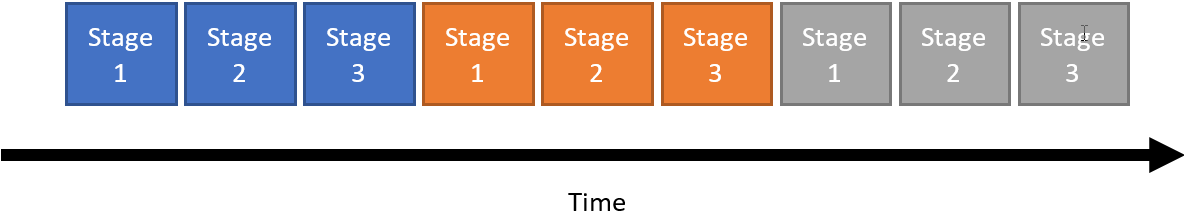ID 683152
Date 12/19/2022
Public

## 5.2.1. Pipeline Loops

Pipelining is a form of parallelization where multiple iterations of a loop execute concurrently, like an assembly line.
Consider the following basic loop with three stages and three iterations. A loop stage is defined as the operations that occur in the loop within one clock cycle.
Figure 29. Basic loop with three stages and three iterationsIf each stage of this loop takes one clock cycle to execute, then this loop has a latency of nine cycles.
The following figure shows the pipelining of the loop from Basic loop with three stages and three iterations.
Figure 30. Pipelined loop with three stages and four iterationsThe pipelined loop has a latency of five clock cycles for three iterations (and six cycles for four iterations), but there is no area tradeoff. During the second clock cycle, Stage 1 of the pipeline loop is processing iteration 2, Stage 2 is processing iteration 1, and Stage 3 is inactive.

This loop is pipelined with a loop initiation interval (II) of 1. An II of 1 means that there is a delay of 1 clock cycle between starting each successive loop iteration.

The Intel® HLS Compiler Pro Edition attempts to pipeline loops by default, and loop pipelining is not subject to the same constant iteration count constraint that loop unrolling is.

Not all loops can be pipelined as well as the loop shown in Pipelined loop with three stages and four iterations, particularly loops where each iteration depends on a value computed in a previous iteration.

For example, consider if Stage 1 of the loop depended on a value computed during Stage 3 of the previous loop iteration. In that case, the second (orange) iteration could not start executing until the first (blue) iteration had reached Stage 3. This type of dependency is called a loop-carried dependency.

In this example, the loop would be pipelined with II=3. Because the II is the same as the latency of a loop iteration, the loop would not actually be pipelined at all. You can estimate the overall latency of a loop with the following equation:

where is the number of cycles the loop takes to execute and is the number of cycles a single loop iteration takes to execute.

The Intel® HLS Compiler Pro Edition supports pipelining nested loops without unrolling inner loops. When calculating the latency of nested loops, apply this formula recursively. This recursion means that having II>1 is more problematic for inner loops than for outer loops. Therefore, algorithms that do most of their work on an inner loop with II=1 still perform well, even if their outer loops have II>1.